# Thermodynamic Features and Design of Solar-Air Source Composite Heating System

Thermodynamic Features and Design of Solar-Air Source Composite Heating System
School of Science, Xi'an University of Architecture and Technology, Xi'an 710055, China

Corresponding Author Email:
nina@xauat.edu.cn
Page:
967-975
|
DOI:
https://doi.org/10.18280/ijht.380424
15 June 2020
|
Accepted:
25 October 2020
|
Published:
31 December 2020
| Citation

OPEN ACCESS

Abstract:

Facing the huge energy consumption of buildings, it is highly practical to study every aspect of energy-saving technologies for heating and cooling devices. Solar thermal technology and air/water source heat pump are two popular energy-saving technologies, which could be combined into a composite heating system with good energy-saving effect and efficiency. This paper constructs a solar-air source composite heating system, analyzes its thermodynamic features, and builds up the corresponding thermodynamic model. In addition, the authors modeled the daytime and nighttime heat balances, as well as the monthly cumulative heat supply for the constructed system. Finally, a dual-tank composite heating system was designed with independent heat supply/storage. The proposed models and system were proved valid and scientific through experiments.

Keywords:

solar heating, air source heat pump (ASHP), thermodynamic feature analysis, composite heating system design

1. Introduction

Currently, buildings take up for about 35% of all energy consumed in China. This proportion increases year by year with the development of society [1-4]. Around 75% of building energy consumption are utilized for winter heating and summer cooling. Therefore, it is highly practical to study every aspect of energy-saving technologies for heating and cooling devices [5, 6].

The relatively mature building energy-saving technologies mainly adopt two strategies for energy-efficient heating: solar thermal technology and air/water source heat pump. The former strategy can solve the peak-valley difference of energy demand through energy storage and release; the latter transfers the thermal energy of low-grade heat sources to high-grade heat sources, so that no high-grade heat source is wasted in building heating [7, 8]. These two strategies could be combined into a composite heating system with good energy-saving effect and efficiency.

Solar thermal technology collects solar energy, and converts it into thermal energy for storage; the stored energy can be utilized by thermal terminals [9-11]. To mitigate the heat loss in convection in light-to-heat conversion, Roumpedakis et al.  developed a novel porous structure installed between the heat-absorbing fin and the cover plate of flat-plate collector, which enhances the heat transfer between heat transfer medium and absorber plate/water. Focusing on flat-plate collector, Zeng et al.  explored the natural convection features between water and heat transfer medium in solar collector tubes of different structures, as well as their heat collection performance, found that the heating system has no requirement on pump consumption, and optimized the thickness of the insulation layer at different angles for the collector tube with a triangular flow channel section. Under constant illumination and supply/return water temperature, Yu et al.  studied how the physical properties of the heat storage body in solar heating system affect the heat storage features and solar load rate, and constructed a mathematical model of the collector aimed to optimize the heat collection per unit area and tilt angle.

In 2001, China launched the coal-to-electricity project. Since then, the air source heat pump (ASHP) has been widely applied to heat supply. Many Chinese scholars have explored the winter energy consumption, heating stability, and heating comfort of this heating method . Nogueira et al.  surveyed the operation of the ASHP heating system in rural areas of northern China, and analyzed the heating performance and use economy of the system under different control strategies. Ong  conducted a Fluent simulation of the actual operating effects and influencing factors of the ASHP radiant floor heating system, and designed an example system based on the calculation of parameters like heating temperature coefficient, house space area, floor material selection, pipe length, and pipe diameter.

In a low temperature environment, the ASHP system performs poorly and consumes too much energy. Meanwhile, the solar thermal system has the problems of unstable heat exchange in light-to-heat conversion, and the mismatch between supply and demand, owing to the instability of solar energy [18-22]. Huan et al.  combined the solar thermal system with the ASHP system into a composite heating system, which improves the energy-saving efficiency of building heating. Many scholars have probed deep into the performance and influencing factors of such a composite heating system. Dombrovsky et al.  built up a mathematical model of the composite heating system, simulated the system based on TRNSYS, and proved the high fitness between the actual and simulated systems. Davies et al.  constructed a transient calculation model for the composite heating system, analyzed the operating economy of the system, calculated the system’s annual expense and return on investment, and optimized the system model based on the calculation results.

This paper thoroughly analyzes the thermodynamic features of the solar-air source composite heating system, and rationalizes the system design. The proposed design could mitigate the mutual interference between solar collector and ASHP, reduce the working time of the ASHP system, and improve the utilization rate of solar heating, resulting in enhanced operating performance of the composite heating system.

The rest of this paper is organized as follows: Section 2 sets up the architecture of the composite heating system, analyzes the thermodynamic features of the system, and establishes the corresponding thermodynamic model; Section 3 models the daytime and nighttime heat balances, as well as the monthly cumulative heat supply for the constructed system; Section 4 gives the steps and keys of the design for a dual-tank composite heating system was designed with independent heat supply/storage; Section 5 verifies the effectiveness and scientific nature of the proposed models and system; Section 6 summarizes the findings of this research.

2. System Architecture and Thermodynamic Features

Figure 1 shows the architecture of a single-tank heating system that combines solar thermal technology and ASHP. There are three thermal cycles in the system: solar collector heat generation cycle, ASHP heat generation cycle, and indoor water heating cycle.

## 1.pngFigure 1. The architecture of a single-tank solar-air source composite heating system

In the solar collector heat generation cycle, the heat collector converts solar energy into thermal energy. Then, the thermal energy is absorbed by the heat absorption layer, and used to heat up the cold water in the heat collection tubes. Since the heat collector is placed with a tilt angle, there is a temperature difference between its inlet and outlet. By the thermosiphon principle, the circulating water pump makes the water to circulate in the system. The cold water from the hot water storage tank is heated, and then flows back to the tank, serving as a heat storage medium that stores the collected solar thermal energy.

In the ASHP heat generation cycle, the evaporator absorbs the low-temperature heat from the air through evaporation. The liquid fluorine medium is vaporized, compressed by the compressor, and converted into a hot high-pressure gas, which then heats up the cold water in the condenser. The hot water returns to the hot water storage tank to store the thermal energy from the hot high-pressure gas. Meanwhile, the fluorine medium gas is uniformly liquefied by depressurization at the expansion valve, producing a cold low-pressure liquid. The liquid is pumped to the evaporator again to kick off the next cycle of gasification and liquefaction.

In the indoor water heating cycle, the hot water in the hot water storage tank is pumped out by the booster water pump to supply the domestic water for the building, and to form a heating cycle in the indoor heating pipes. The hot water flows out from the tank towards the building to supply heat to the interior; then, the cold water flows back to the tank, and heated up by solar energy and hot high-pressure gas again.

Before thermodynamic feature analysis, the following assumptions were made for the solar-air source composite heating system: the composite heating system receives solar energy, air energy and electric energy, and outputs the thermal energy of the hot water in the hot water storage tank.

When the composite heating system outputs heat to the building, the output thermal energy is positive; when the composite heating system absorbs heat from the outside, the output thermal energy is negative. Let CW and MFW be the constant pressure specific heat and mass flow of the circulating water flow in the composite heating system, respectively. Then, the output heat of the system can be expressed as:

$H={{C}_{p}}\cdot M{{F}_{W}}\cdot ({{t}_{out}}-{{t}_{in}})$       (1)

where, tin and tout are the inlet and outlet temperatures of the water tank, respectively; Tin and Tout are the inlet and outlet thermodynamic temperatures of the water tank, respectively; TR is the room temperature. The thermal exergy output can be expressed as:

$EX{{E}_{H}}={{C}_{W}}\cdot M{{F}_{W}}\cdot [({{T}_{out}}-{{T}_{in}})-{{T}_{R}}\cdot \ln (\frac{{{T}_{out}}}{{{T}_{in}}})]$           (2)

The electric input to the system can be expressed as:

$E={{P}_{C}}-(H-{{P}_{SE}})/{{\mu }_{ASP}}$       (3)

where, PH is the thermal power output of the composite heating system; PSE is the effective heat collection of the solar collector; PC is the power consumption of the voltage drop device; μASP is the heat generation performance coefficient of the ASHP. The exergy input of the system can be can be expressed as:

$EX{{E}_{E}}=E={{P}_{C}}-(H-{{P}_{SE}})/{{\mu }_{ASP}}$       (4)

The total energy output and total exergy output by the system can be respectively expressed as:

$W=H+E$     (5)

$EXE=EX{{E}_{H}}+EX{{E}_{E}}$     (6)

The total heating coefficient λtotal, heating coefficient λH, and power consumption coefficient λE of the composite heating system can be expressed as:

$\left\{ \begin{matrix} {{\lambda }_{total}}=\frac{W}{{{P}_{SE-total}}} \\ {{\lambda }_{H}}=\frac{H}{{{P}_{SE-total}}} \\ {{\lambda }_{E}}=\frac{E}{{{P}_{SE-total}}} \\ \end{matrix} \right.\text{ }$            (7)

where, PSE-total is the total heat collection of the solar collector. The total exergy efficiency γtotal, thermal exergy efficiency γH, and electric exergy efficiency γE of the composite heating system can be expressed as:

$\left\{ \begin{matrix} {{\lambda }_{total}}=\frac{EXE}{EX{{E}_{SE-total}}} \\ {{\lambda }_{H}}=\frac{EX{{E}_{H}}}{EX{{E}_{SE-total}}} \\ {{\lambda }_{E}}=\frac{EX{{E}_{E}}}{EX{{E}_{SE-total}}} \\ \end{matrix} \right.\text{ }$                  (8)

where, EXESE is the total exergy of the solar collector. Taking the surface temperature of the sun as 5,770°C, the EXESE value can be calculated by:

\begin{align} & EX{{E}_{SE-total}} \\ & ={{P}_{SE-total}}\cdot [1-\frac{5}{4}\cdot (\frac{{{T}_{R}}}{5770})+\frac{1}{4}\cdot {{(\frac{{{T}_{R}}}{5770})}^{4}}] \\ \end{align}              (9)

3. Heat Balance Modeling

According to the working principle of the solar-air source composite heating system, the operating process of the system can be divided into three steps: heat generation, heat storage, and heat supply. In the heat generation step, the heating mode is adjusted according to the lighting conditions. In daytime, the system can switch between single and dual heating modes freely. In nighttime, the ASHP heating mode must be adopted. In the heat storage step, the hot water storage tank stores and supplies heat. The heat balances between system modules can be analyzed based on the operating process of the system during the day and night. In the heat supply step, the hot water storage tank supplies building users with domestic hot water and provides heat to indoor heating cycle. This section mainly models the heat balances of the system at daytime and nighttime.

3.1 Daytime system model

## 2.png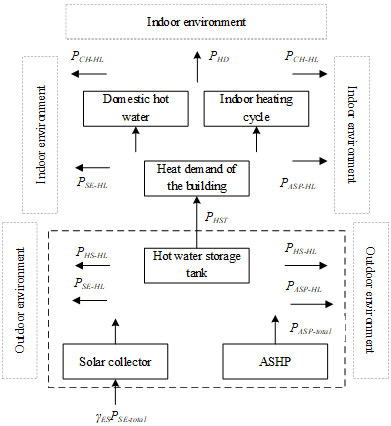Figure 2. The heat balances at daytime

In the daytime, the heat balances of two stages, namely, heat generation-heat storage and heat storage-heat supply, were modeled. Figure 2 shows the heat balances at daytime. For simplicity, the unit time in the transient heat balances was fixed to 1s. Then, the heat balance of heat generation-heat storage can be described by:

\begin{align} & {{P}_{SE-total}}+{{P}_{ASP-total}}-{{P}_{HS-HL}} \\ & -{{P}_{SE-HL}}-{{P}_{ASP-HL}}={{P}_{HST}} \\ \end{align}         (10)

where, PASP-total and PSE-total are the total heat generations of the ASHP system and the solar thermal system, respectively; PSE-HL and PASP-HL are the heat losses in the circulation pipes of the ASHP system and the solar thermal system, respectively; PHST and PHS-HL are the heat output and heat loss of the hot water storage tank, respectively. The heat generation of the solar collector can be calculated by:

${{P}_{SE-P}}={{P}_{SE-total}}{{\delta }_{L-H}}-{{P}_{SE-HL}}$         (11)

where, δL-H is the light-heat conversion efficiency of the solar collector. The heat balance in the heat storage-heat supply can be described as:

${{P}_{HST}}-{{P}_{HS\text{-}HL}}-{{P}_{CH-HL}}={{P}_{HD}}$         (12)

where, PCH-HL is the heat loss in the circulation pipes during heat supply; PHS-HL is the heat loss of the hot water storage tank during heat storage; PHD is the total heat demand of the building. In daytime, the solar thermal system serves as the primary heat source of heat generation-heat storage.

During heat generation-heat storage, the total solar energy acts as a heat source. The light-heat conversion efficiency of the solar collector δL-H depends on the inlet and outlet temperatures of the collector and solar radiation intensity. The correlation of δL-H with the inlet and outlet temperatures and room temperature can be characterized by the normalized temperature difference:

$\Delta {{T}_{in}}=({{T}_{in}}-{{T}_{R}})/SRI$         (13)

where, SRI is the total solar radiation power on the photosensitive surface of the solar collector. For the polypropylene random copolymer (PPR) water pipes, its heat loss is determined by the heat transfer coefficient ηP. During heat generation-heat storage, the heat loss per unit of circulation pipe of the solar collector can be calculated by:

${{P}_{SE-HL}}={{\eta }_{P}}{{h}_{P}}\Delta {{T}_{P}}$        (14)

where, hP is the thickness of the water pipe; ∆TP is the temperature difference between the inner and outer walls of the water pipe. Let ρ be the density of water, and SP be the cross-sectional area of the water pipe. During heat generation-heat storage, the heat loss per unit of circulation pipe of the ASHP can be calculated by:

${{P}_{ASP-HL}}={{C}_{W}}\rho S{}_{p}{{v}_{W}}({{T}_{ASP-out}}-{{T}_{ASP-in}})$         (15)

where, TASP-in and TASP-out are the inlet and output water temperatures of the ASHP, respectively. During heat supply, the total heat demand of the building PHD covers the domestic hot water of building users and the indoor heating load:

${{P}_{HD}}={{P}_{HW}}+{{P}_{CH}}$       (16)

where, PHW is the heat of domestic hot water; PCH is the heat demand for indoor heating. If PHST=PHD, the composite heating system can fully satisfy the heat demand of the building. The PHS-HL value depends on the thermal conductivity ηKW of the insulation material used in the hot water storage tank:

${{P}_{HS-HL}}={{\eta }_{KW}}{{h}_{KW}}\Delta {{T}_{HS}}$       (17)

where, hKW is the thickness of the insulation material; ∆THS is the difference between water temperature of the hot water storage tank and the indoor temperature.

3.2 Nighttime system model

Figure 3 shows the heat balances of the composite heating system at nighttime. During the night, the heat source of heat generation-heat storage is the heat stored in the hot water storage tank and the heat generated by the ASHP. The former can be calculated from the water temperature and water storage in the tank, while the latter can be derived from the inlet-outlet temperature difference of the AHSP. The heat balances at nighttime can be described by:

${{P}_{HS-total}}+{{P}_{ASP-total}}-{{P}_{HS-HL}}-{{P}_{ASP-HL}}={{P}_{HST}}$        (18)

where, PHS-total is the total heat of the hot water in the tank. The heat generation and heat loss in circulation pipes of the ASHP at nighttime were calculated by the same method as in Subsection 3.1; the parameters of heat balances during heat supply were also computed by the same method as in the preceding subsection.

## 3.png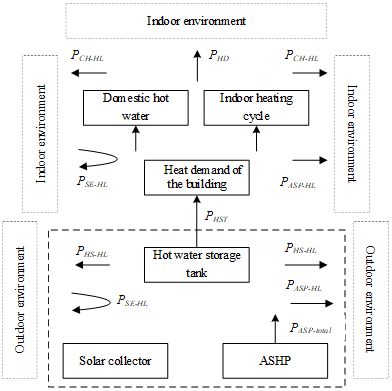Figure 3. The heat balances at nighttime

3.3 Cumulative heat supply in different months

With the change of season, the PASP-total and PSE-total of the composite heating system will vary, so will the PHD. In winter, the PHD value surges up, the mean water temperature in the system declines, and the domestic hot water and indoor heating load both increase. The PHD value drops in spring and autumn, and minimizes in summer (when the system only needs to supply domestic hot water). To evaluate the macro operation performance of the system, the cumulative heat supply was modeled for different seasons.

In spring, summer, and autumn, the per-capita daily demand for hot water was set to 50L. It was assumed that the system supplies hot water of 40°C, and each household has three persons. Then, the cumulative heat supply of the composite heating system can be calculated by:

${{P}_{HSC-O}}=50\cdot 3\cdot {{C}_{W}}\rho (40-\Delta {{T}_{M}})$       (19)

where, ΔTM is the difference between the water temperature in the current month and the annual mean water temperature.

In winter, the cumulative heat supply of the composite heating system can be calculated by adding up the PHSC-O, the cumulative heat supply of the solar collector PSE-D, and the cumulative heat supply of the ASHP PASP-D:

${{P}_{HSC-W}}={{P}_{HSC-O}}+{{P}_{SE-D}}+{{P}_{ASP-D}}$       (20)

4. System Design

Figure 4 illustrates the architecture of our dual-tank composite heating system. The system adopts the independent heat supply/storage mode of solar collector and ASHP, making it easier and more flexible to switch between different heating modes.

## 4.png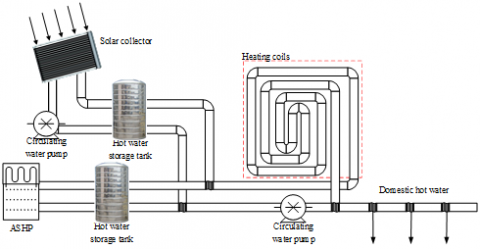Figure 4. The architecture of dual-tank composite heating system

4.1 Heat collection area

The heat collection area determines the heat generation capacity of the solar thermal system. For each kind of solar heat exchange method, there is a unique way to compute the heat collection area. If the solar thermal system adopts the direct heat exchange mode (centralized storage of solar radiation heat in hot water storage tank), the heat collection area SD of solar collector can be calculated by:

${{S}_{D}}=\frac{86400{{P}_{HD}}{{L}_{ES}}}{SRI\cdot {{\gamma }_{ES}}(1-{{\gamma }_{ES-HL}})}$        (21)

where, LES is the load rate of solar thermal system; γES and γES-HL are the mean collection efficiency and heat loss in circulation pipes of the solar collector, respectively.

Table 1 lists the amount of solar radiation and solar load rate in regions of China with different levels of solar energy abundance. From formula (21), it can be learned that the SD value of the solar thermal system with direct heat exchange is mainly affected by the total heat demand of the building, the local solar radiation intensity, the heat load distribution of the solar thermal system, the heat collection efficiency of the collector, and the heat loss in the circulation pipes.

Table 1. The amount of solar radiation and solar load rate in regions of China with different levels of solar energy abundance

 Level of solar energy abundance Amount of solar radiation per unit area LES 1 ≥7,500 70~80 2 6,500~7,500 55~70 3 5,500~6,500 45~55 4 4,500~5,500 30~45 5 ≤4,500 25~30

If the solar thermal system adopts the indirect heat exchange mode, the cold water can be heated up by the solar radiation heat, using a plate heat exchanger. The different working media on the two sides of the exchanger might lead to temperature difference. To prevent this temperature difference, the heat collection area SI must be relatively large. Let ηES be the total heat loss coefficient of the solar collector, and SHS and ηHS be the heat exchange area and heat transfer coefficient of the heat exchanger in the hot water storage tank, respectively. Then, the SI value can be computed by:

${{S}_{I}}={{S}_{D}}(1+\frac{{{\eta }_{ES}}{{S}_{D}}}{{{\eta }_{HS}}{{S}_{HS}}})$      (22)

Let FES-U be the flow of heat transfer medium per unit area of the collector’s cross-sectional area. Then, the circulation flow FES-C of the heat transfer medium in the solar thermal system can be calculated by:

${{F}_{ES\text{-}C}}={{F}_{ES\text{-}U}}{{S}_{ES}}$       (23)

where, SES is the heat collection area of the solar collector in the solar thermal system. If the system adopts the direct heat exchange mode, S=SD; if the system adopts the indirect heat exchange mode, S=SI. Table 2 provides the flows of common heat transfer media per unit area.

Table 2. The flows of common heat transfer media per unit area

 Type of solar thermal systems FES-U Solar water heater Vacuum tube 0.041~0.078 Flat plate 0.075 Direct centralized solar heating 0.019~0.057 Small solar heating 0.022~0.041 Indirect centralized solar heating 0.013~0.017 Composite heating system 0.036

4.2 ASHP model

To satisfy the maximum heat demand of the building, the heat generation on the nameplate of the ASHP is the transient value tested under standard working conditions, without considering the heat loss caused by defrosting. Under the actual working conditions in cold regions or in winter, the actual heat generation PASP-T of the composite heating system can be calculated by:

${{P}_{ASP-T}}={{P}_{ASP-total}}\times {{\eta }_{DB}}\times {{\eta }_{DE}}$        (24)

where, ηDB and ηDE are the correction coefficients of dry bulb and defrosting temperatures, respectively. The common values of ηDB are listed in Table 3.

Table 3. The common values of defrosting temperatures

 Dry-bulb temperature Defrosting temperature -8 0.70 -6 0.74 -4 0.77 -2 0.80 0 0.83 2 0.89 4 0.92 6 0.96 8 1.01

4.3 Tank volume

The heating performance of the composite heating system hinges on the volume of the hot water storage tank. Suppose the vertical temperature change of the water flow in the tank is uniform, with a negligible heat loss throughout the heating process. Then, the tank volume VES-HS for the solar thermal system can be calculated by:

${{V}_{ES-HS}}={{S}_{ES}}\cdot {{V}_{ES-HS-U}}$        (25)

where, VES-HS-U is the tank volume corresponding to each unit of heat collection area in the solar thermal system. As shown in formula (25), the tank volume for the solar thermal system is constrained by the following factors: the heat collection area, installation budget, and installation position of the collector. Table 4 presents the tank volumes corresponding to each unit of heat collection area for heating systems with different heat storage modes.

Table 4. The tank volumes of different heating systems

 Type of thermal heat storage Tank volume corresponding to each unit of heat collection area Domestic hot water 60-110 Short-term storage 60-160 Long-term storage 1,500-2,200

In the ASHP heating system, the tank volume is influenced by the circulating water capacity and the duration of defrosting in cold regions or in winter. Suppose the defrosting period tDE lasts 5min, the maximum decline in hot water temperature ΔTmax is 3°C, and the total length of coils per unit area LASP is 5m. Then, the tank volume can be computed by:

\begin{align} & {{V}_{ASP-HS}}=\frac{{{P}_{ASP-T}}\times {{t}_{DE}}}{{{C}_{W}}\times \Delta {{T}_{\max }}}-0.15\times {{L}_{ASP}} \\ & =\frac{180{{P}_{ASP-T}}}{3{{C}_{W}}}-0.15\times {{L}_{ASP}} \\ \end{align}               (26)

5. Experimental Verification

This section attempts to verify whether the design of the proposed composite heating system, and its thermodynamic models are scientific. Firstly, the composite heating system was applied in a high and cold region. The variation in solar radiation intensity and indoor temperature during the heating process was recorded (Figure 5). It can be seen that, on each day, the solar radiation intensity peaked at around 12:00, and the indoor temperature reached the maximum at about 16:00.

To improve the heating performance, the heating ratio of the two heat sources in the system must be reasonably regulated from period to period. Therefore, the inlet and outlet water temperatures of the water tank, and transient solar radiation intensity during the heat generation of the composite heating system were simulated. Part of the data were exported from the solar collector heat generation cycle, ASHP heat generation cycle, and indoor water heating cycle, respectively. Then, the calculated values and measured values were imported to the established thermodynamic model and heat balance models for verification.

The calculated and measured total heat demands of the building at daytime and nighttime are compared in Figures 6(a) and 6(b), respectively. Obviously, the time lag of hot water supply in the water tank increased the error in the total heat demand of the building.

## 5a.png(a)

## 5b.png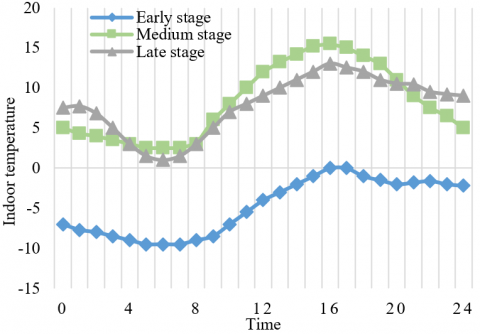(b)

Figure 5. The variation in solar radiation intensity (a) and indoor temperature (b) during the heating process

## 6a.png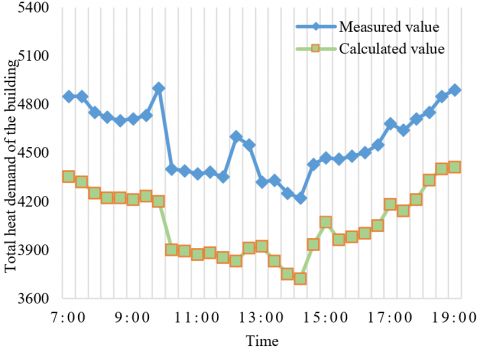(a)

## 6b.png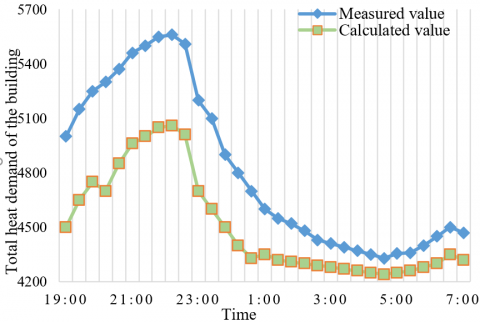(b)

Figure 6. The total heat demand of the building at daytime (a) and nighttime (b)

Figure 7 provides the normalized temperature difference of solar collector at daytime. It can be seen that the normalized temperature difference was fitted well, with a mean relative error of 6.49%. The above results show that the heat balance model of daytime applies to the thermodynamic analysis of the heating system in high and cold regions.

## 7.png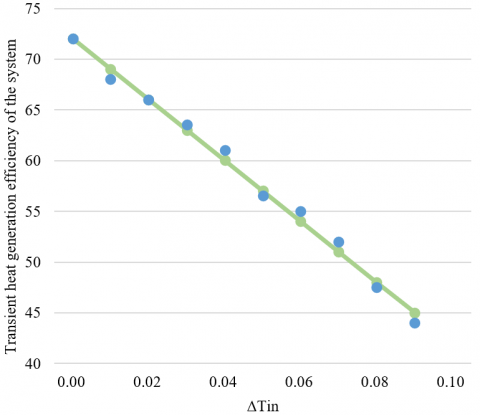Figure 7. The normalized temperature difference of solar collector at daytime

Figure 8 displays the heat generation performance of ASHP heating system at different target temperatures of heat generation. It can be seen that, for the ASHP heating system, with the decline in indoor temperature, the ratio of the heat generation to the shaft power of the compressor under the same working condition gradually decreased. In other words, the ASHP heating system has a low efficiency and high energy waste, when the room temperature is low and the target temperature of heat generation in high. This is consistent with the actual situation.

## 8.png## 8.pngFigure 8. The variation in heat generation performance of ASHP heating system

The ASHP heating system could work continuously at nighttime and on rainy/snowy days. The heat generation of the system under these conditions is recorded in Figure 9. It can be seen that the heat generation at nighttime changed similarly as that on rainy/snowy days, and the relative error at nighttime followed basically the same trend as that on rainy/snowy days. With the extension of heat generation period, the heat generation had a slight decline. Frost phenomenon exerted a great impact on the system. Low ASHP automatically defrosts during about 120-160min. Increasing the defrost frequency can reduce the heat generation and relative error.

Figure 10 compares the relative error between heat generation and heat storage of the composite heating system in different periods at nighttime. During The night, the mean relative error of the system’s heat generation stood at 7.91%, suggesting that the heat balance of the system is greatly influenced by the ambient temperature at night.

## 9.pngFigure 9. The heat generation of the ASHP heating system at nighttime and on rainy/snowy days

## 10.pngFigure 10. The relative error between heat generation and heat storage in different periods at nighttime

## 11.pngFigure 11. The heat generation of solar thermal system and ASHP heating system

Figure 11 provides the heat generation curves of the two heat sources of the composite heating system at daytime. It can be seen that a good heat generation effect was achieved through the collaboration between solar thermal system and ASHP heating system. The overall effect was desirable, despite a certain time lag in the assistance of ASHP. In a few periods, the solar radiation was blocked by the clouds. In this case, the actual heat generation of the system decreased with the inlet water temperature of the collector. Hence, the heat balance did not change gradually in this scenario, resulting in a slight rise in relative error from 5.74% to 13.42%.

Figure 12 compares the relative error between heat generation and heat storage of the system in each month. The relative error was negative in the cold winter, indicating that the calculated total heat demand of the building is smaller than the actual value. In other months, the relative error was positive, that is, the calculated total heat demand of the building is greater than the actual value. These findings agree well with the actual situation.

## 12.pngFigure 12. The relative error between heat generation and heat storage in each month

6. Conclusions

In this paper, a solar-air source composite heating system is established for thermodynamic feature analysis and thermodynamic modeling. The heat balances of the composite heating system were modeled for different periods in daytime and nighttime. Besides, the cumulative heat supply of the system was modeled for different months. Then, the authors designed a dual-tank composite heating system with independent heat supply/storage, and specified the steps and key points in the design. Experimental results show that the proposed models are effective and scientific, and basically in line with the actual situation.

Acknowledgment

Natural Science Special Fund of Xi'an University of Architecture and Technology ZR19056. A Special Research Program of Shaanxi Provincial Department of Education 19JK0460 funded by the Provincial Natural Science Foundation 2019JQ-766.

References

 Poppi, S., Bales, C., Heinz, A., Hengel, F., Chèze, D., Mojic, I., Cialani, C. (2016). Analysis of system improvements in solar thermal and air source heat pump combisystems. Applied Energy, 173: 606-623. https://doi.org/10.1016/j.apenergy.2016.04.048

 Yerdesh, Y., Abdulina, Z., Aliuly, A., Belyayev, Y., Mohanraj, M., Kaltayev, A. (2020). Numerical simulation on solar collector and cascade heat pump combi water heating systems in Kazakhstan climates. Renewable Energy, 145: 1222-1234. https://doi.org/10.1016/j.renene.2019.06.102

 Song, M., Deng, S., Mao, N., Ye, X. (2016). An experimental study on defrosting performance for an air source heat pump unit with a horizontally installed multi-circuit outdoor coil. Applied Energy, 165: 371-382. https://doi.org/10.1016/j.apenergy.2015.12.107

 Panagiotidou, M., Aye, L., Rismanchi, B. (2020). Solar driven water heating systems for medium-rise residential buildings in urban Mediterranean areas. Renewable Energy, 147: 556-569. https://doi.org/10.1016/j.renene.2019.09.020

 Allaerts, K., Coomans, M., Salenbien, R. (2015). Hybrid ground-source heat pump system with active air source regeneration. Energy Conversion and Management, 90: 230-237. https://doi.org/10.1016/j.enconman.2014.11.009

 Mattinen, M.K., Nissinen, A., Hyysalo, S., Juntunen, J.K. (2015). Energy use and greenhouse gas emissions of air-source heat pump and innovative ground-source air heat pump in a cold climate. Journal of Industrial Ecology, 19(1): 61-70. https://doi.org/10.1111/jiec.12166

 Hakkaki-Fard, A., Aidoun, Z., Ouzzane, M. (2015). Improving cold climate air-source heat pump performance with refrigerant mixtures. Applied Thermal Engineering, 78: 695-703. https://doi.org/10.1016/j.applthermaleng.2014.11.036

 Safa, A.A., Fung, A.S., Kumar, R. (2015). Comparative thermal performances of a ground source heat pump and a variable capacity air source heat pump systems for sustainable houses. Applied Thermal Engineering, 81: 279-287. https://doi.org/10.1016/j.applthermaleng.2015.02.039

 Biglarian, H., Saidi, M.H., Abbaspour, M. (2019). Economic and environmental assessment of a solar-assisted ground source heat pump system in a heating-dominated climate. International Journal of Environmental Science and Technology, 16(7): 3091-3098. https://doi.org/10.1007/s13762-018-1673-3

 Hamdan, M.A., Aboushi, A.R.A., Abdelhafez, E.A. (2014). Solar thermal hybrid heating system. Jordan Journal of Mechanical and Industrial Engineering, 11(3): 181-184.

 Engel, G., Asenbeck, S., Koell, R., Kerskes, H., Wagner, W., van Helden, W. (2017). Simulation of a seasonal, solar-driven sorption storage heating system. Journal of Energy Storage, 13: 40-47. https://doi.org/10.1016/j.est.2017.06.001

 Roumpedakis, T.C., Kallis, G., Magiri-Skouloudi, D., Grimekis, D., Karellas, S. (2020). Life cycle analysis of ZEOSOL solar cooling and heating system. Renewable Energy, 154: 82-98. https://doi.org/10.1016/j.renene.2020.02.114

 Zeng, J., Li, N.P.,Cheng, J.L., Zhang, Y., Wang, C. (2016). Evaluation of the sensible heat storage air source heat pump for residential heating in central-south China. Energy Procedia, 88: 703-708. https://doi.org/10.1016/j.egypro.2016.06.047

 Yu, S.F., Nan, X.H., Liang, K. (2016). Analytical solution and its experimental validation of water temperature of air-source heat pump. Acta Energiae Solaris Sinica, 37(8): 2026-2031.

 El, E., Çakmak, G., Arguhan, Z., Yıldız, C. (2015). Experimental investigation of solar stills integrated with solar water heating collectors. Journal of Thermal Science and Technology, 37(2): 97-107.

 Nogueira, C.E.C., Vidotto, M.L., Toniazzo, F., Debastiani, G. (2016). Software for designing solar water heating systems. Renewable and Sustainable Energy Reviews, 58: 361-375. https://doi.org/10.1016/j.rser.2015.12.346

 Ong, K.S. (2016). Review of solar, heat pipe and thermoelectric hybrid systems for power generation and heating. International Journal of Low-Carbon Technologies, 11(4): 460-465. https://doi.org/10.1093/ijlct/ctv022

 Andrade, L.A., Barrozo, M.A.S., Vieira, L.G.M. (2016). A study on dynamic heating in solar dish concentrators. Renewable Energy, 87: 501-508. https://doi.org/10.1016/j.renene.2015.10.055

 Dong, J.K., Li, L., Jiang, Y.Q., Tian, H., Tian, Y., Hu, W.J. (2016). Effects oftwo kinds of phase change materials heat exchanger on energe storage performance of multi-split air source heat pump. Acta Energiae Solaris Sinica, 37(11): 2856-2861.

 Ni, L., Qv, D., Yao, Y., Niu, F., Hu, W. (2016). An experimental study on performance enhancement of a PCM based solar-assisted air source heat pump system under cooling modes. Applied Thermal Engineering, 100: 434-452. https://doi.org/10.1016/j.applthermaleng.2016.02.001

 Shan, M., Yu, T.H., Yang, X. (2016). Assessment of an integrated active solar and air-source heat pump water heating system operated within a passive house in a cold climate zone. Renewable Energy, 87: 1059-1066. https://doi.org/10.1016/j.renene.2015.09.024

 Cruz, T., Schaeffer, R., Lucena, A.F., Melo, S., Dutra, R. (2020). Solar water heating technical-economic potential in the household sector in Brazil. Renewable Energy, 146: 1618-1639. https://doi.org/10.1016/j.renene.2019.06.085

 Huan, C., Wang, F., Li, S., Zhao, Y., Liu, L., Wang, Z., Ji, C. (2019). A performance comparison of serial and parallel solar‐assisted heat pump heating systems in Xi'an, China. Energy Science & Engineering, 7(4): 1379-1393. https://doi.org/10.1002/ese3.357

 Dombrovsky, L.A., Kokhanovsky, A.A., Randrianalisoa, J.H. (2019). On snowpack heating by solar radiation: a computational model. Journal of Quantitative Spectroscopy and Radiative Transfer, 227: 72-85. https://doi.org/10.1016/j.jqsrt.2019.02.004

 Davies, G., Blower, J., Hall, R., Maidment, G. (2020). Preliminary study of a solar assisted heating system. In ASHRAE Winter Conference, 126: 39-47. https://www.ashrae.org/conferences/2020-winter-conference-orlando.